# Catch up on the latest AI articles# Teach Augment: Optimizing Data Augmentation By Utilizing Teacher Models

3 main points
✔️ Proposed a data augmentation strategy Teach Augment, which introduces a supervised model to adversarial data augmentation and does not require careful parameter adjustment
✔️ Proposed a data augmentation strategy using a neural network that combines a model for data augmentation on color and a model for data augmentation on geometric transformations
✔️Confirmed that the proposed method outperforms existing data augmentation search frameworks, including state-of-the-art methods, for image classification and semantic segmentation tasks without adjusting hyperparameters for each task

written by Teppei Suzuki
(Submitted on 25 Feb 2022 (v1), last revised 28 Mar 2022 (this version, v3))

Subjects: Computer Vision and Pattern Recognition (cs.CV)code：The images used in this article are from the paper, the introductory slides, or were created based on them.

## first of all

Recently, data augmentation has attracted much attention as an important method for model generalization.
As a prior work on such data augmentation, AutoAugment has been proposed to automatically search for efficient augmentation methods for model generalization.
This method is based on an adversarial strategy that searches for extension methods that maximize the task loss of the target model and is known to improve model generalization.

However, as shown in the left side of Fig. 1, adversarial data augmentation can be unstable without constraints, since loss maximization is achieved by collapsing the intrinsic meaning of the image. To avoid this happening, conventional methods regularize the augmentation based on empirical rules or restrict the search range of the size parameter of the function in the search space, but the problem is that many parameters need to be tuned.

To solve this problem, an online data augmentation optimization method TeachAugment is proposed in this paper, which uses teacher knowledge.
TeachAugment is also based on adversarial data augmentation, but as shown on the right side of Fig. 1, it can explore augmentation methods to the extent that the transformed image is recognizable for the teacher model.
Also, unlike conventional adversarial data augmentation, TeachAugment does not require any prior distribution or hyperparameters, thus avoiding excessive augmentation methods that would destroy the original meaning of the image.
As a result, Teach Augment does not require parameter tuning to ensure that the transformed image is recognizable.

Furthermore, in this paper, a data augmentation method using neural networks with two functions: data augmentation on geometric transformations and data augmentation on colors is proposed. This method can represent most of the functions in the search space of AutoAugment and its composite functions with only two transformations.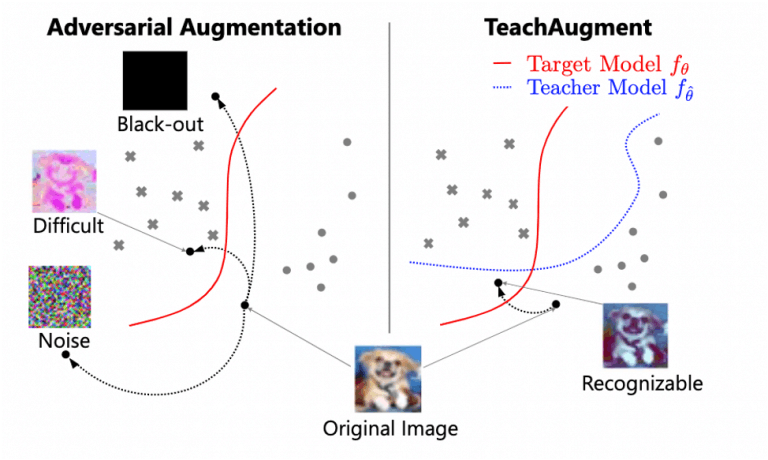Fig. 1: Difference between conventional and proposed methods

The contributions of this paper are as follows.

• We propose an online data augmentation optimization framework based on adversarial strategies using teacher knowledge called TeachAugment.
TeachAugment can make adversarial data augmentation strategies more profitable without carefully tuning parameters by using teacher models to ensure that the inherent meaning of images is not corrupted.
• We also propose a neural network-based data augmentation method.
This method simplifies the design of the search space and the parameters can be updated by TeachAugment's gradient method.
• We show that TeachAugment outperforms traditional methods such as online data augmentation and state-of-the-art augmentation strategies on classification, semi-integration, and unsupervised representation learning tasks without adjusting hyper-parameters or search space size for each task.

In the subsequent chapters, we will briefly explain data augmentation and adversarial as prior knowledge, and then describe the proposed method, experimental details, and results.

## What is Data Extension?

Data expansion is a method to increase the number of data by applying some transformation to the image to simulate the image as shown in Figure 2.
There are countless types of transformations other than those shown in Fig. 2, such as Erasing, which fills a part of the image, and GaussianBlur, which applies a Gaussian filter.

Models trained with these various extension methods are usually known to be more generalizable than models trained only on the original data, but sometimes they can degrade performance or induce unexpected biases.
Therefore, to improve the generalization rate of a model, we need to manually find effective data extension methods based on the domain.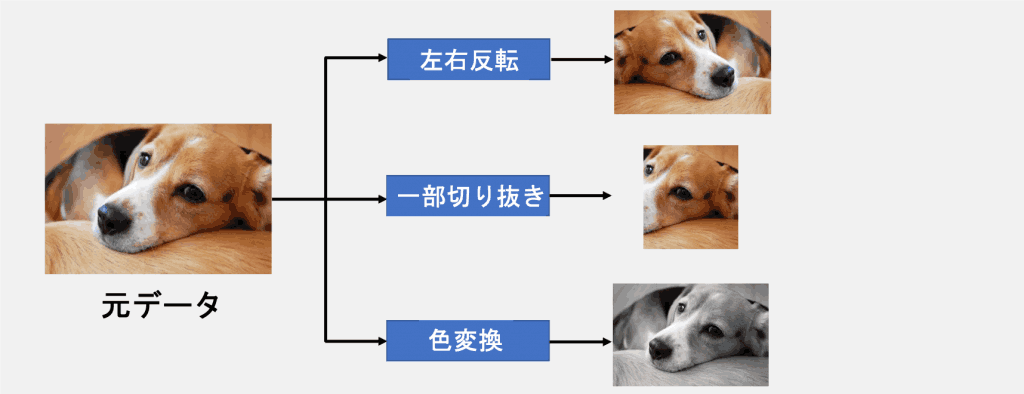Figure 2: Data Extension Overview

## proposed method

In this paper, we propose two data augmentation strategies called Teach Augment and a data augmentation method using neural nets.

### Teach Augment

Before going into the description of the first proposal, Teach Augment, we briefly discuss general learning with data augmentation and adversarial data augmentation.
The letters that appear in each expression are defined as follows.

• $X \sim \mathcal{X}$:$\mathcal{X}$ sampled image from $\mathcal{X}$.
• Data extension function with $a_\phi$:$\phi$ as a parameter
• $\phi$:Neural net parameters
• $f_\theta$:target model
• $f_{\hat{\theta}}$:Teacher model

### General learning with data augmentation

The mini-batch sample $x$ is transformed by inputting it into the data extension function $a_\phi$, which is then input into the target network $f_{\theta}$. ($f_\theta\left(a_\phi(x)\right)$)

The parameters of the target model are then updated to minimize the loss $L$ by SGD using the following equation

$\min _\theta \mathbb{E}_{x \sim \mathcal{X}}} L\left(f_\theta\left(a_\phi(x)\right)\right.$

### hostile data augmentation

In the adversarial data extension, to improve the generalizability of the model, we search for the parameter $\phi$ that maximizes the loss of the target model, as in the following equation

$\max _\phi \min _\theta \mathbb{E}_{x \sim \mathcal{X}}} L\left(f_\theta\left(a_\phi(x)\right)\right.$

### Teach Augment

As mentioned in the introduction, in adversarial data expansion, the feature (meaning) of the image itself may be lost by maximizing $\phi$, and to cope with such a problem, we have conventionally set restrictions such as regularization and limiting the search space.
However, in this paper, we propose a data expansion strategy that eliminates the need for such restrictions by using a supervised model.

The data augmentation strategy according to the following formula is called TeachAugment.

$\max _\phi \min _\theta \mathbb{E}_{x \sim \mathcal{X}}\left[L\left(f_{\theta}}\left(a_\phi(x)\right)\right)-L\left(f_{\hat{\theta}}\left(a_\phi(x)\right)\right)\right]$

If the transformed image is too far away from the original image, the second term $L\left(f_{\hat{\theta}}\left(a_\phi(x)\right)\right)$ becomes large, which is negative for the overall maximization problem and prevents such transformation methods from being chosen.

This equation can be solved by alternately updating $a_\phi$ and $f_\theta$ in SGD (stochastic gradient descent) as shown in Figure 3.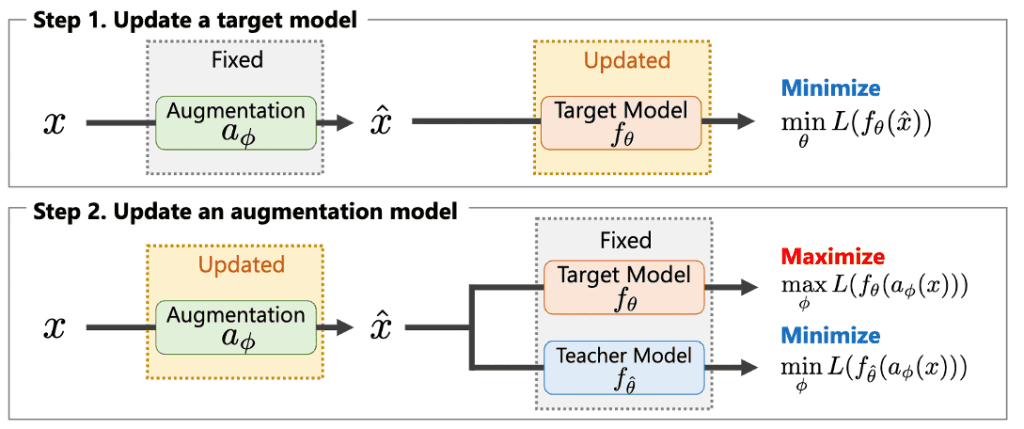Figure 3: Parameter update algorithm

Also, the gradient of the first term in the Teach Augment formula often saturates in the maximization problem concerning $\phi$ if the target model is very accurate.
To prevent this, we set the usual loss function with cross-entropy $\sum_{k=1}^K-y_k \log f_\theta\left(a_\phi(x)\right)_k$, but this time $\sum_{k=1}^K y_k \log \left(1-f_\theta\left( a_\phi(x)\right)_k\right)$ (non-saturated loss).

### Data augmentation using neural nets

The second proposal, a data expansion method using neural networks, is described.
Here, data expansion is performed using neural network $a_{\phi$, which combines model $c_{\phi_c}$ for data expansion on color and model $g_{\phi_g}$ for data expansion on geometric transformation.
The letter that appears in each expression is defined as follows.

• $c_{\phi_c}$:Model for color data extension
• $g_{\phi_g}$: model for data expansion on geometric transformations
• $a_\phi=g_{\phi_a} \circ c_{\phi_c}$:$c_{\phi_c}$ and $g_{\phi_g}$ combined neural net (synthetic function of $c_{\phi_c}$ and $g_{\phi_g}$)
• $\phi=\{\phi_c,\phi_g\}$:neural net parameters(set of $\phi_c$ and $\phi_g$)
• $x \in \mathbb{R}^{M \times 3}$: Image before transformation ($M$ is the number of pixels, 3 corresponds to RGB)
• $\tilde{x}_i \in [0,1]$:color extended image
• $\alpha_i, \beta_i \in \mathbb{R}^3$: scale and shift parameters
• $z~\mathcal{N(0,I_N)}$: N-dimensional Gaussian distribution with mean 0 and variance $I_N$.
• $c$: one-hot correct labels in image classification, omitted for other tasks
• $t(\dot)$:Triangular wave($t(x)=\arccos (\cos (x \pi)) / \pi$)
• $A \in \mathbb{R}^{2 \times 3}$:Residual parameters
• $I \in \mathbb{R}^{2 \times 3}$:unit matrix

The following formula defines the data extension for color

$\tilde{x}_i=t\left(\alpha_i \odot x_i+\beta_i\right),\left(\alpha_i, \beta_i\right)=c_{\phi_c}\left(x_i, z, c\right)$

The model $c_{\phi_c}$, which performs data expansion on color, can in principle transform an input image into an arbitrary image if the model size is large enough, due to the universal approximation theorem.

The following equation then defines the data extension for geometric transformations

$\hat{x}=\operatorname{Affine}(\tilde{x}, A+I), A=g_{\phi_g}(z, c)$

where $\operatorname{Affine}(\tilde{x}, A+I)$ means to affine transform (translate, scale, rotate) $\tilde{x}$ with parameter $A+I$.

## experimental setup

We evaluated our method on an image classification task to compare it with the existing data augmentation method search.

We trained WideResNet-40-2 (WRN-40-2), WideResNet-28-10 (WRN-28-10), Shake-Shake (26 2×96d), PyramidNet with ShakeDrop regularization, and ResNet-50 for ImageNet.

In addition to the above image classification tasks, we evaluated our method using semantic segmentation.
For semantic segmentation, we trained FCN-32s, PSPNet, and Deeplabv3 on Cityscapes.

## Results and Discussion

### Evaluation of the objective function

We evaluate the effectiveness of our proposed objective function (the formula introduced in TeachAugment).
As a baseline, we replaced the objective function of our framework with the loss of adversarial autoaugment (Adv. AA) and point augment (PA) for comparison.
To make a fair comparison, we performed the experiments under all the same conditions except for the objective function.
The results of the error rate (%) under each condition are shown in Figure 4.

We can see that the error rate of Adv.AA has decreased from the baseline.
We speculate that this is because the model performing data expansion produces unrecognizable images and confuses the target model.
Also, in the case of Adv. AA, the size of the search space needs to be carefully adjusted to guarantee convergence, but there is no such restriction in the proposed method.

We can confirm that our method can achieve a better error rate than PointAugment and performs the best among the three.
In PointAugment, the upper limit of loss for the extended data is controlled by dynamic parameters, but the proposed method has no such restriction.
As a result, we believe that our method achieves more diverse transformations than PointAugment and improves the error.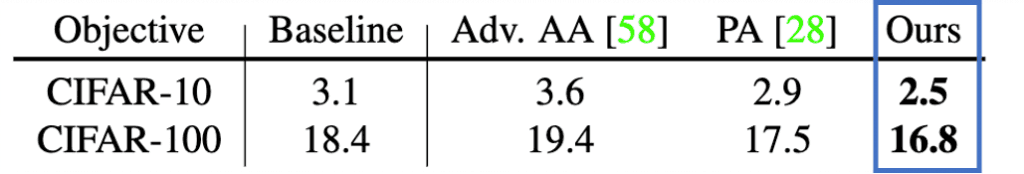Figure 4: Objective function evaluation results

### image classification

To confirm the effectiveness of our method, we compared it with the prior methods AutoAugment (AA), PBA, Fast AA, FasterAA, DADA, RandAugment (RandAug), OnlineAugment (OnlineAug), Adv. AA.

The error rate (%) results for each condition are shown in Figure 5.
We observed that our method achieved the same error rate as the other methods, except for Adv.AA, which uses multiple extended samples per mini-batch.
In particular, ImageNet achieves the lowest error rate percentage among the methods that do not use multiple extension methods.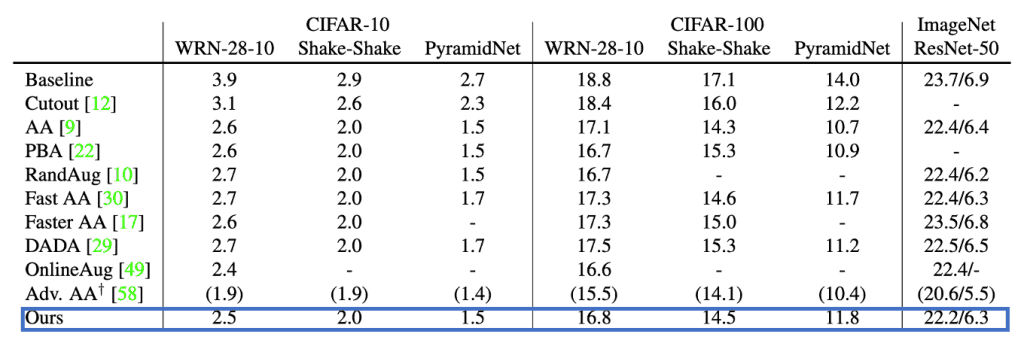Figure 5: Error rate for image classification task

### semantic segmentation

We also evaluated our method using Cityscapes.
We used widely used models: the ResNet-101 backbone, FCN-32s, PSPNet, and Deeplabv3.
We also employed RandAugment and TrivialAugment as baseline methods.
Similar to the proposed plans, these methods are also characterized by the fact that they have few hyperparameters and do not require careful parameter tuning.

The mIoU results for each condition are shown in Fig. 5.
We can see that our method achieves the best mIoU for each model.

We can confirm that RandAugment worsens the IoU of FCN-32s and TrivialAugment fails to improve the mIoU for any model.
Previous studies have reported that TrivialAugment does not perform well on tasks other than image classification.
The reason for the poor accuracy of RandAugment and TrivialAugment is that these two search spaces are not suitable for the semantic segmentation task and model capacity the semantic segmentation task and the capacity of the model.
However, the proposed method improves mIoU in all conditions without adjusting the parameters from the classification task, which confirms the effectiveness of the proposed method.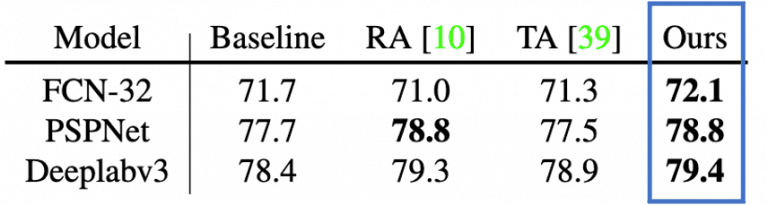Figure 6: mIoU results for the semantic segmentation task.

## summary

In this paper, an online data augmentation optimization method called TeachAugment is proposed, which introduces a supervised model to adversarial data augmentation and performs more informative data augmentation without requiring careful parameter tuning. The paper is available at:
We have also proposed a method for data augmentation using neural nets that combine models for data augmentation on color and models for data augmentation on geometric transformations.

Experimental results show that the proposed method outperforms existing data augmentation and search frameworks including state-of-the-art methods for image classification and semantic segmentation tasks without adjusting the hyper-parameters of each task.

If you have any suggestions for improvement of the content of the article,